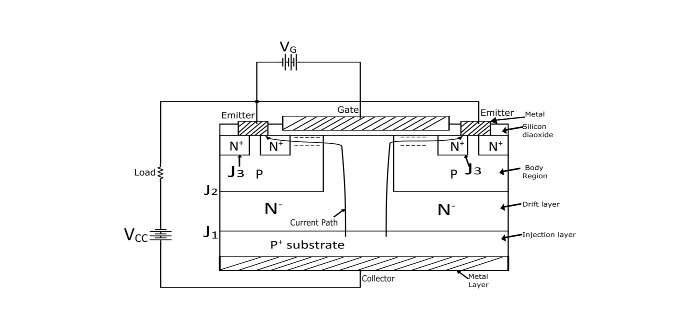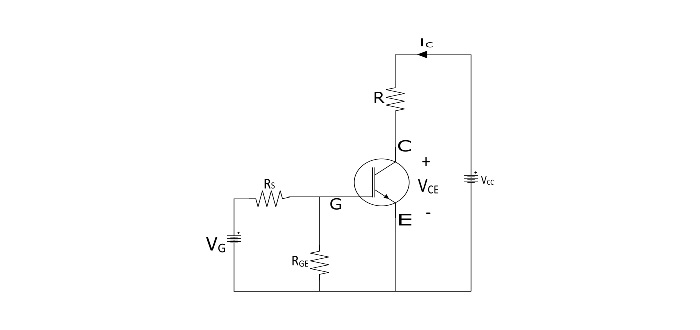# Insulated Gate Bipolar Transistor Operation and Characteristics

The Insulated Gate Bipolar Transistor (IGBT) is a semiconductor device having three terminals – Gate (G), Emitter (E), and Collector (C). IGBT has been developed by combining the best qualities of both BJT and Power MOSFET. Hence, an IGBT exhibits high input impedance as a PMOSFET and has low ON-state power losses like a BJT.

The IGBT is also free from the second breakdown problem that present in the BJT.

IGBT is also known as Insulated Gate Transistor (IGT), Metal Oxide Insulated Gate Transistor (MOSIGT), Conductively Modulated Field Effect Transistor (COMFET) or Gain Modulated FET (GEMFET).

## Basic Structure and Schematic Symbol of IGBT

The IGBT is constructed by a p+ layer substrate from the collector. In the IGBT, the p+ substrate is called the injection layer since it injects holes into the N- layer (in between the P+ layer and P layer) is called drift region. The thickness of N- layer determines the voltage blocking capability of the IGBT. The P-layer is known as the body region of the IGBT and the body region serves to accommodate depletion of junction J2.## Working of IGBT

When collector is made positive with respect to the emitter, the IGBT is forward biased. When voltage is not applied between gate and emitter, the junction J2 between N- region and P region is reverse biased, hence no current flows from collector to emitter.

When gate is applied with positive voltage with respect to emitter by source VG, with gate-emitter voltage greater than threshold voltage (VGET) of the IGBT, then an n-channel or inversion layer is induced in the upper part of p-region just below the gate. This n-channel short circuits the N--region with the N+-emitter regions.

Electrons start to flow from N+ region to N- region through the n-channel. Since the IGBT is forward biased, hence the P+ collector region injects holes into the N- drift region, so that the injection carrier density in the N- drift region increases extensively and in turn, the conductivity of N- region increases significantly. Hence the IGBT gets turned ON and starts to conduct forward collector current IC.

The collector current (IC) or emitter current (IE) consists of two components viz. one is Hole Current (Ih) due to the injected holes and another is Electron Current (Ie) due to injected electrons. Hence,

The collector or load current 𝐼𝐶 = emitter currentIC=Ih+Ie

Since the major component of the collector current is due to the electron current (Ie). Hence,

$$\mathrm{I_{c} \cong I_{E}}$$

The ON state voltage drop in IGBT is,

VCE.on = Voltage Drop[in−channel +across drift in N− region +across forward biased P+ N junction J1]

## Output or Static I-V Characteristics of IGBT

The static I-V characteristics or output characteristics of IGBE is the graph between the collector current (IC) and the collector-emitter voltage (VCE) plotted for various values of gate-emitter voltage.

The forward output characteristics is similar to that of BJT. The voltage VRM is the maximum reverse breakdown voltage.## Transfer Characteristics of IGBT

The transfer characteristics of IGBT is the graph between the collector current (IC) and gate-emitter voltage (VGE). When the value of VGE is smaller than threshold voltage (VGET), the IGBT remains in the OFF state.• Lower gate-driver requirements
• Lower switching losses
• Smaller snubber circuit requirement
• Less size and cost
• High efficiency

## Applications of IGBT

• IGBT are used in dc and ac motor drives
• Used in UPS systems
• Used in power supplies and drivers for solenoids, relays and contactors